# Hypergeometrical Universe

## The Hypergeometrical Force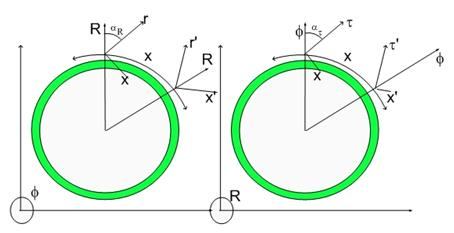The Hypergeometrical Force

When I DERIVED the equation for the Hypergeometrical Force – the Grand Unification Force - used a setup with two infinitesimal elements of current (or mass flow).

These infinitesimal elements of current or flow defined a direction within the four dimensional spatial manifold. This direction is shown as velocities in the formulas.  Despite of having velocities, these formulas were derived for constant velocities, where the velocities were referred from a relaxed Fabric of Space reference system.  To eliminate this constraint one has to derived the potential through adiabatic integration followed by the derivation of a generalized force according to Lagrangian formalism!!!

These means that the force equations below are constant velocity forces. Despite of this constraint, they are quite usefull for making simplied reasonings about dynamics.

Here are the equations for Electromagnetism and Gravitation: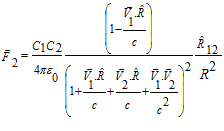(1)

Where C1 and C2 are the charges traveling at V1 and V2 and c is the speed of light. R12 is a vector from charge 1 to charge 2.

Similarly for Gravitation: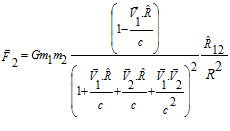Notice that the only difference is the proportionality coefficient. This means that the interaction is the same – dilaton field-dilator interaction controlled by the Quantum Lagrangian Principle. The proportionality coefficient ratio is given by the ratio of these two angles a0 and a1:R0 is the age of the Universe times the speed of light (15.82 billion light years) and λ1 is the Compton wavelength of a Hydrogen atom. To be precise, this ratio has to be multiplied by an elastic coefficient of the Fabric of Space. This coefficient is the reason why dilaton and Gravitational waves exist.

The humongous (1036) factor is easy to understand by this simple figure. A more detailed analysis can be found in the pdf containing the theory.

This equation can be simplified for the case where there is no rotation and one of the bodies is much larger than the other (Sun and Mercury for instance) into: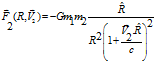This is the first time a force is actually derived from a purely geometrical theory of the Universe, so it took me some time to understand that this equation has to be integrated upon R (distance) to yield the amount of energy that can be shared into Kinetic and Potential at any position.

Adiabatic integration upon R yields the potential(3)

This potential is the one used in the Precession of Mercury's Perihelion problem. Applying it to Lagrangian Formalism yields the more appropriate force formula for physical bodies or charges:

Similarly for F one obtains: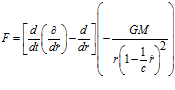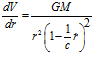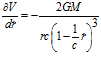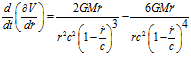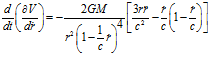Thus F becomes: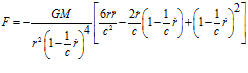Expanding it in powers of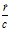Neglecting terms above the second order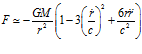This means that my Gravitational Force does not contains first order dependence upon v/c and also that it perfectly describes all celestial dynamics. It produces the same formula for the Precession of Mercury or Earth or anything else Perihelion…JIn summary, my formula produces not one but TWO formulas for force. One for constructs that cannot change speed (e.g. Light) and another for bodies. They are reproduced without approximations below: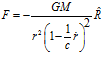The second formula is used to derive the Gravitational Lensinng (Gerber failed to do so since he only ansatz (guessed) right the first formula).

The more complex formulation for the Force per unit mass (for double jets Black Holes or White Orifices) is given by: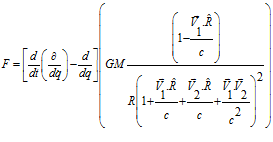To understand the rest of the Universe, one only need to add the Fundamental Dilator paradigm.

The Fundamental Dilator is a coherence between two deformation states within the 4D spatial manifold. The orientation of the letters in the figure below maps the orientation of the dilator deformation with respect to our 3D Hypersurface. A vertical lettering means that the dilator overlaps minimally with our 3DUniverse (the Fundamental Dilator is quite flat (along the fourth dimension).

The Fundamental Dilator can be represented by this diagram:

## Mercury's Perihelion Prequel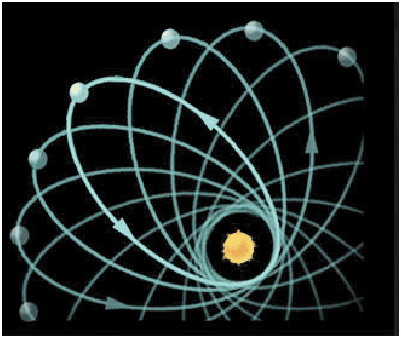## Inflation Theory and Energy Conservation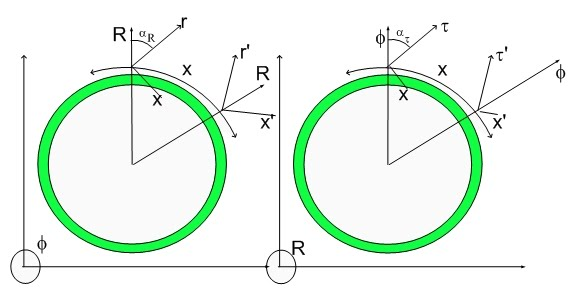Inflation Theory and Energy Conservation

or
RSS FeedCreate your own visitor map